Examples

Chapter 15 Class 10 Probability (Term 1)
Serial order wise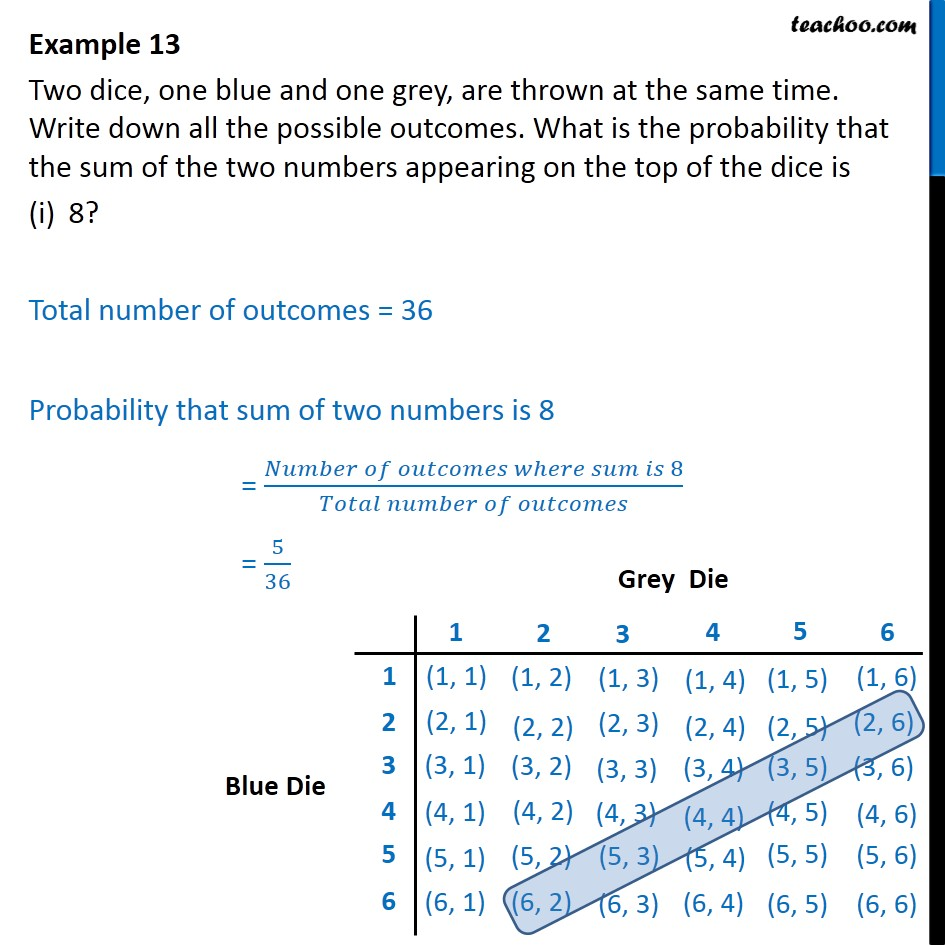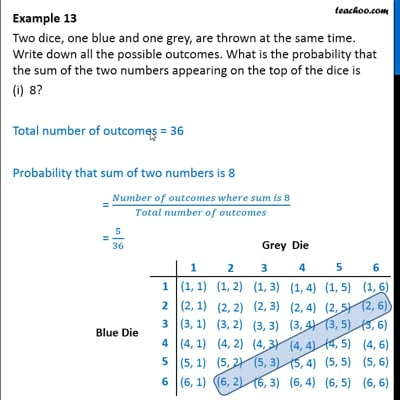This video is only available for Teachoo black users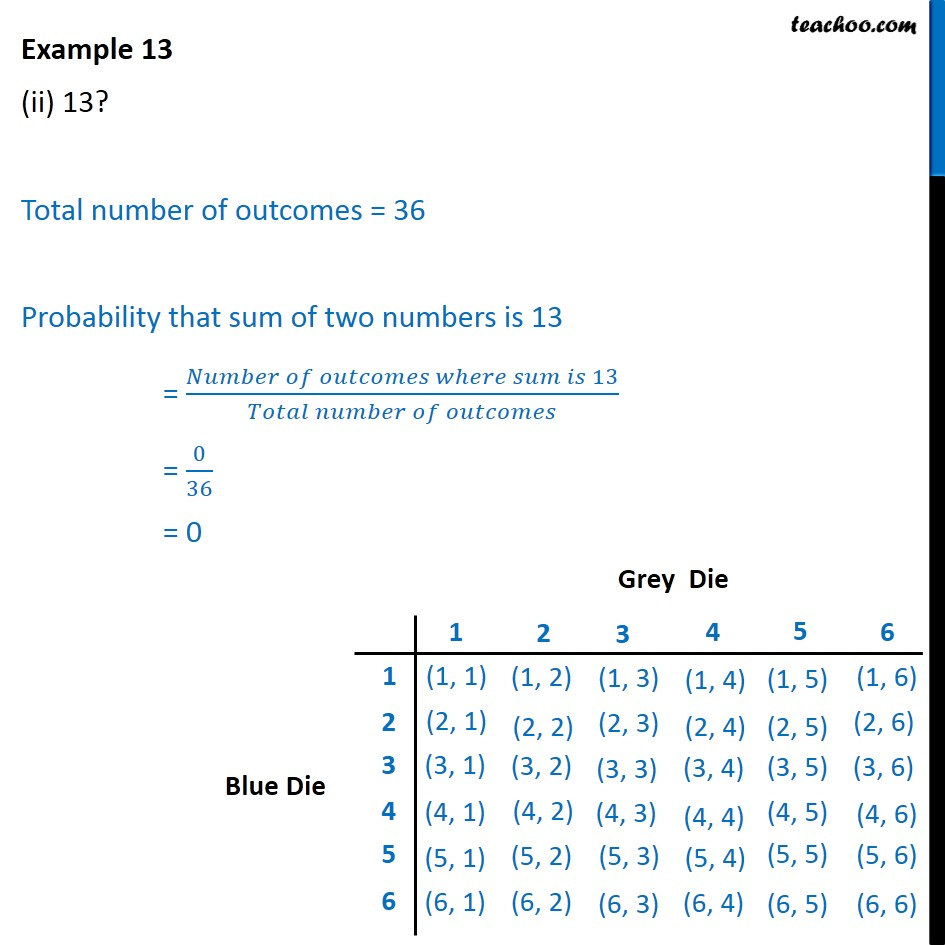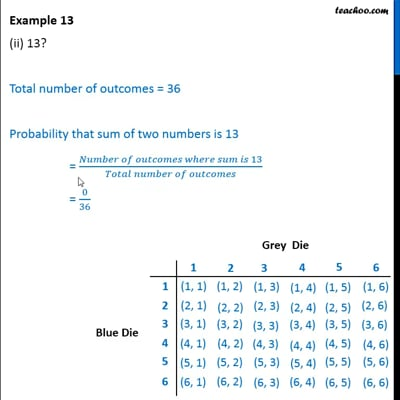This video is only available for Teachoo black users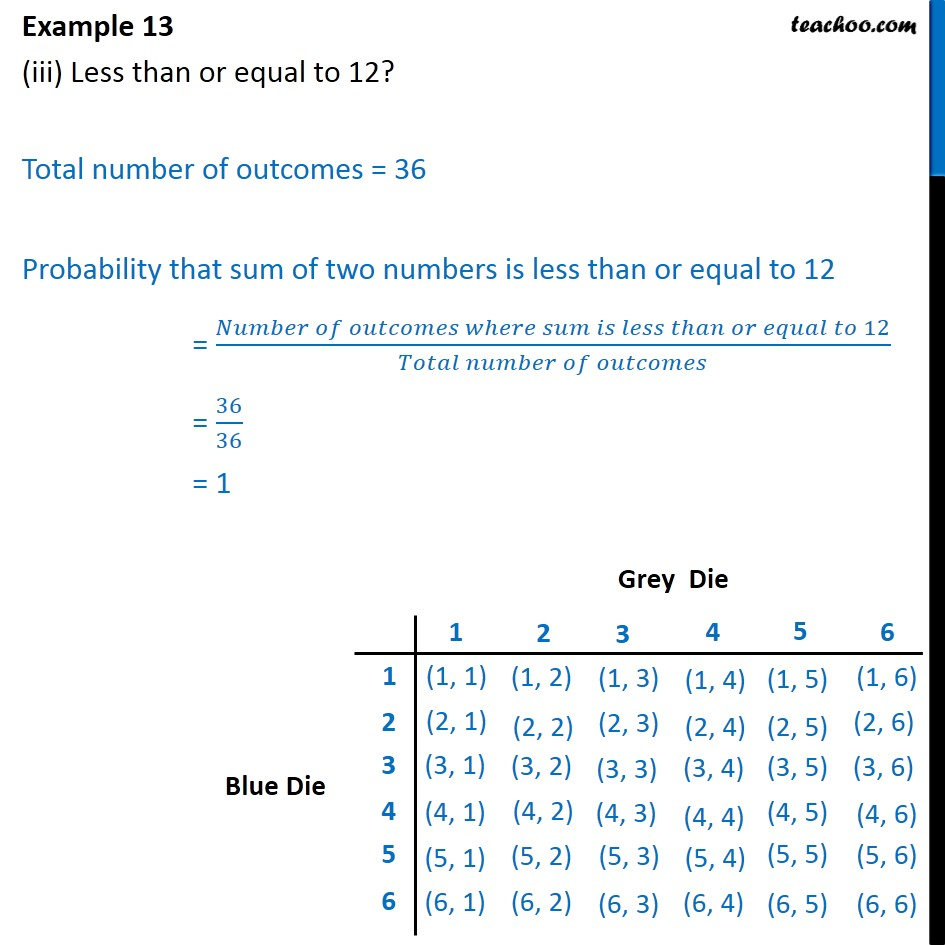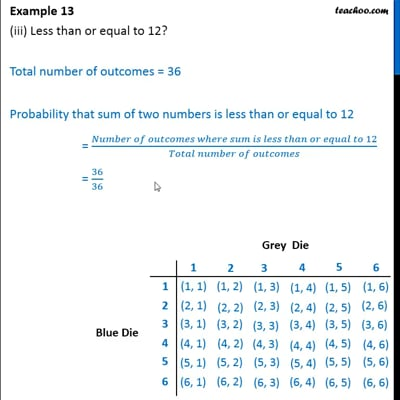This video is only available for Teachoo black users

### Transcript

Example 13 Two dice, one blue and one grey, are thrown at the same time. Write down all the possible outcomes. What is the probability that the sum of the two numbers appearing on the top of the dice is 8? Total number of outcomes = 36 Probability that sum of two numbers is 8 = (𝑁𝑢𝑚𝑏𝑒𝑟 𝑜𝑓 𝑜𝑢𝑡𝑐𝑜𝑚𝑒𝑠 𝑤ℎ𝑒𝑟𝑒 𝑠𝑢𝑚 𝑖𝑠 8)/(𝑇𝑜𝑡𝑎𝑙 𝑛𝑢𝑚𝑏𝑒𝑟 𝑜𝑓 𝑜𝑢𝑡𝑐𝑜𝑚𝑒𝑠) = 5/36 Example 13 (ii) 13? Total number of outcomes = 36 Probability that sum of two numbers is 13 = (𝑁𝑢𝑚𝑏𝑒𝑟 𝑜𝑓 𝑜𝑢𝑡𝑐𝑜𝑚𝑒𝑠 𝑤ℎ𝑒𝑟𝑒 𝑠𝑢𝑚 𝑖𝑠 13)/(𝑇𝑜𝑡𝑎𝑙 𝑛𝑢𝑚𝑏𝑒𝑟 𝑜𝑓 𝑜𝑢𝑡𝑐𝑜𝑚𝑒𝑠) = 0/36 = 0 Example 13 (iii) Less than or equal to 12? Total number of outcomes = 36 Probability that sum of two numbers is less than or equal to 12 = (𝑁𝑢𝑚𝑏𝑒𝑟 𝑜𝑓 𝑜𝑢𝑡𝑐𝑜𝑚𝑒𝑠 𝑤ℎ𝑒𝑟𝑒 𝑠𝑢𝑚 𝑖𝑠 𝑙𝑒𝑠𝑠 𝑡ℎ𝑎𝑛 𝑜𝑟 𝑒𝑞𝑢𝑎𝑙 𝑡𝑜 12)/(𝑇𝑜𝑡𝑎𝑙 𝑛𝑢𝑚𝑏𝑒𝑟 𝑜𝑓 𝑜𝑢𝑡𝑐𝑜𝑚𝑒𝑠) = 36/36 = 1